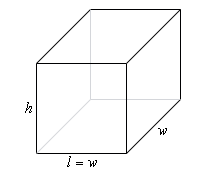Paul's Online Notes
Home / Calculus I / Applications of Derivatives / Optimization
Show Mobile Notice Show All Notes Hide All Notes
Mobile Notice
You appear to be on a device with a "narrow" screen width (i.e. you are probably on a mobile phone). Due to the nature of the mathematics on this site it is best views in landscape mode. If your device is not in landscape mode many of the equations will run off the side of your device (should be able to scroll to see them) and some of the menu items will be cut off due to the narrow screen width.

### Section 4.8 : Optimization

5. We have 45 m2 of material to build a box with a square base and no top. Determine the dimensions of the box that will maximize the enclosed volume.

Show All Steps Hide All Steps

Start Solution

The first step is to do a quick sketch of the problem. We could probably skip the sketch in this case, but that is a really bad habit to get into. For many of these problems a sketch is really convenient and it can be used to help us keep track of some of the important information in the problem and to “define” variables for the problem.

Here is the sketch for this problem.Show Step 2

Next, we need to set up the constraint and equation that we are being asked to optimize.

We are told that we have 45 m2 of material to build the box and so that is the constraint. The amount of material that we need to build the box is then,

$45 = lw + 2\left( {lh} \right) + 2\left( {wh} \right) = {w^2} + 2wh + 2wh = {w^2} + 4wh$

Note that because there is no top the first term won’t have the 2 that the second and third term have. Be careful with this kind of thing it is easy to miss if you aren’t paying attention.

We are being asked to maximize the volume so that equation is,

$V = lwh = {w^2}h$

Note as well that we went ahead and used fact that $$l = w$$ in both of these equations to reduce the three variables in the equation down to two variables.

Show Step 3

Now, let’s solve the constraint for $$h$$ (that will allow us to avoid dealing with roots, plus there is only one $$h$$ in the constraint so it will simply be easier to deal with).

$h = \frac{{45 - {w^2}}}{{4w}}$

Plugging this into the volume formula gives,

$V\left( w \right) = {w^2}\left( {\frac{{45 - {w^2}}}{{4w}}} \right) = \frac{1}{4}w\left( {45 - {w^2}} \right) = \frac{1}{4}\left( {45w - {w^3}} \right)$ Show Step 4

Finding the critical point(s) for this shouldn’t be too difficult at this point so here is that work.

$V'\left( w \right) = {\frac{1}{4}}\left( {45 - 3{w^2}} \right)\hspace{0.5in} \to \hspace{0.5in}\,{\frac{1}{4}}\left( {45 - 3{w^2}} \right) = 0\hspace{0.5in} \to \hspace{0.5in}w = \pm \sqrt {\frac{45}{3}} = \pm \sqrt {15}$

Because we are dealing with the dimensions of a box the negative width doesn’t make any sense and so the only critical point that we can use here is : $$w = \sqrt {15}$$.

Be careful here and do not get into the habit of just eliminating the negative values. The only reason for eliminating it in this case is for physical reasons. If we had just given the equations without any physical reasoning it would have to be included in the rest of the work!

Show Step 5

The second derivative of the volume function is,

$V''\left( w \right) = - \frac{3}{2}w$

From this we can see that the second derivative is always negative for positive $$w$$ (which we will always have for this case since $$w$$ is the width of a box). Therefore, provided $$w$$ is positive, $$V\left( w \right)$$ will always be concave down and so the single critical point we got in Step 4 must be a relative maximum and hence must be the value that gives a maximum volume.

Show Step 6

Now, let’s finish the problem by getting the remaining dimensions.

$l = w = \sqrt {15} = 3.8730\hspace{0.5in}h = \frac{{45 - 15}}{{4\sqrt {15} }} = 1.9365$

The final dimensions are then,

$\require{bbox} \bbox[2pt,border:1px solid black]{{l = w = 3.8730\hspace{0.5in}h = 1.9365}}$